# NCERT Solutions for Class 8 Maths Chapter 5 Exercise 5.2

NCERT Solutions for Class 8 Maths Chapter 5 Exercise 5.2 – Data Handling, has been designed by the NCERT to test the knowledge of the student on the topic – Circle Graph or Pie Chart – Drawing pie charts

### NCERT Solutions for Class 8 Maths Chapter 5 Exercise 5.2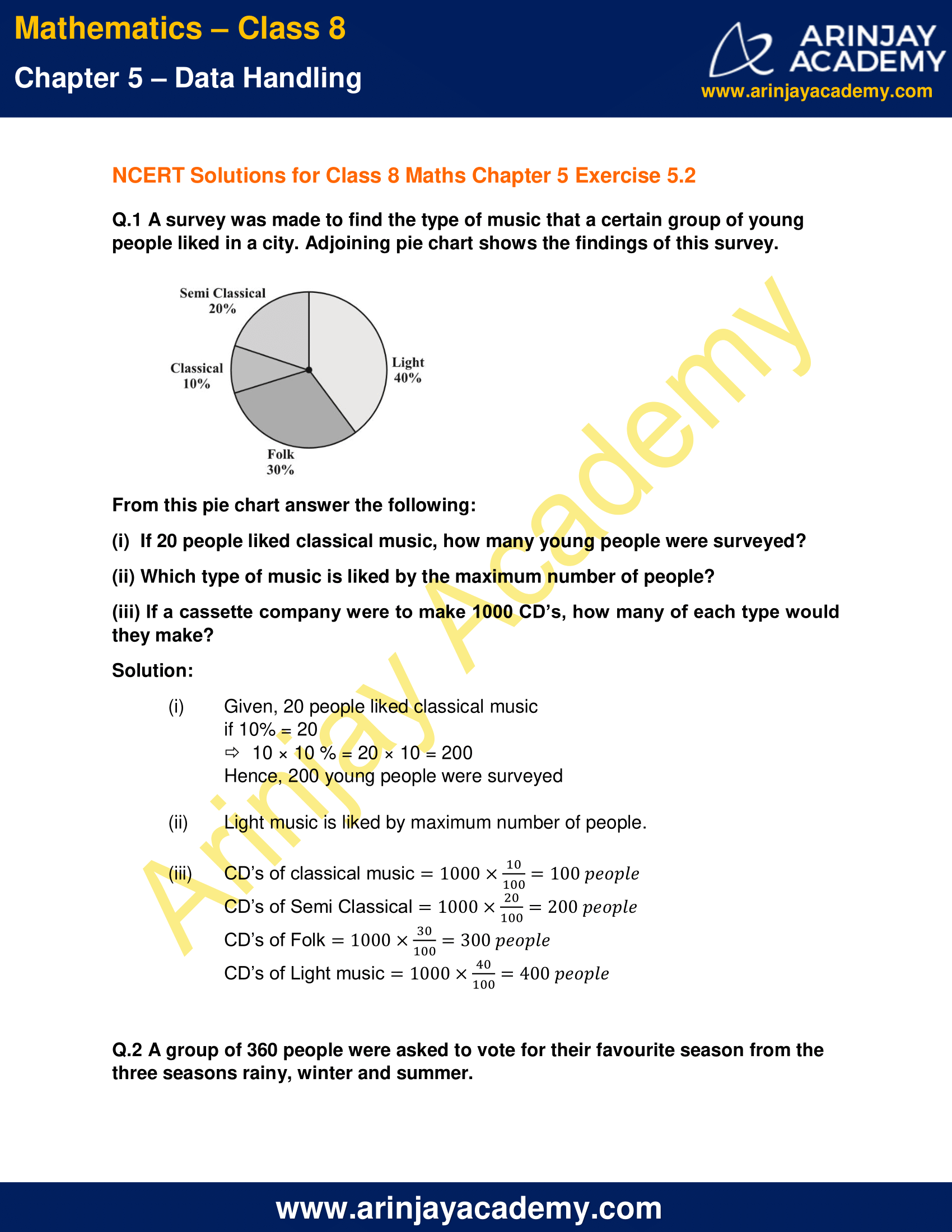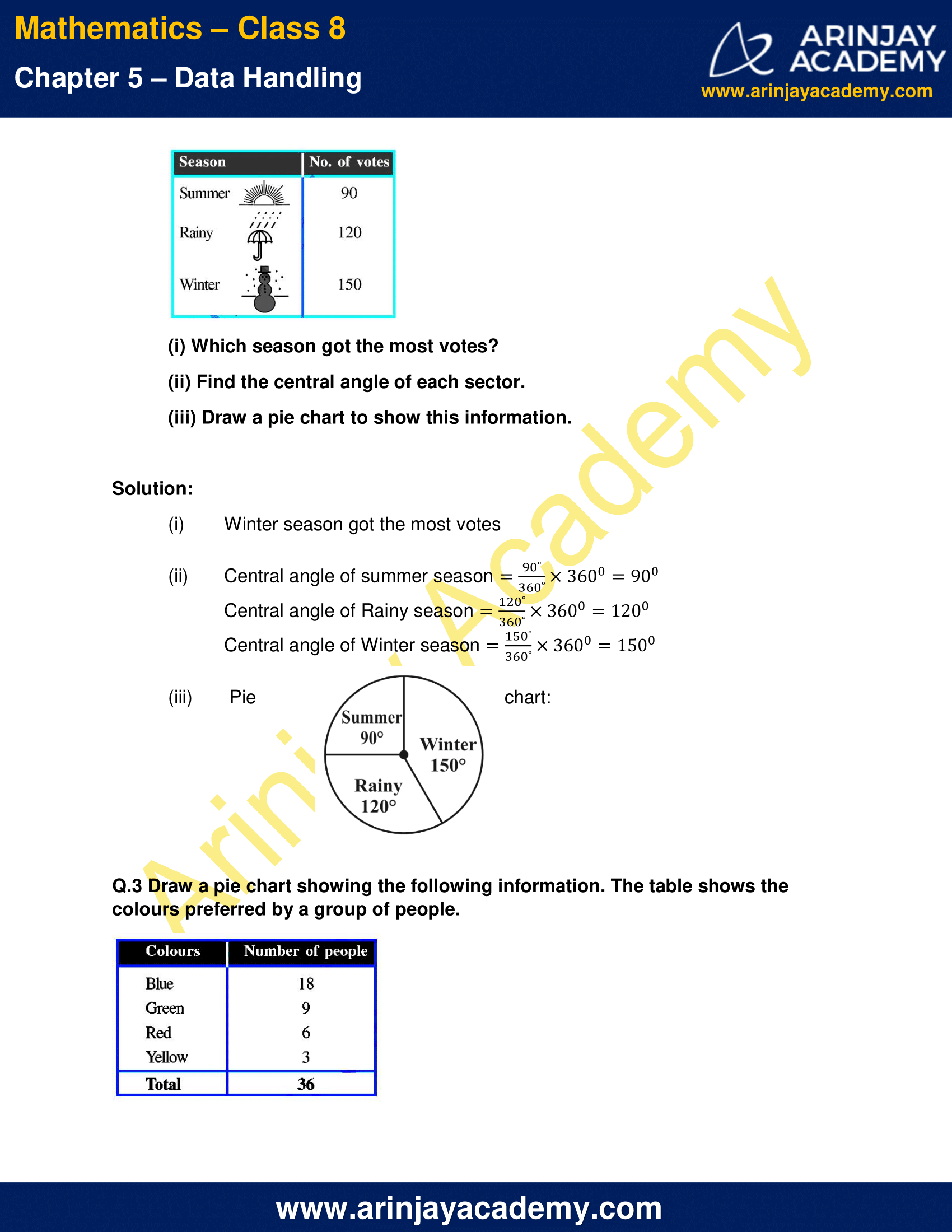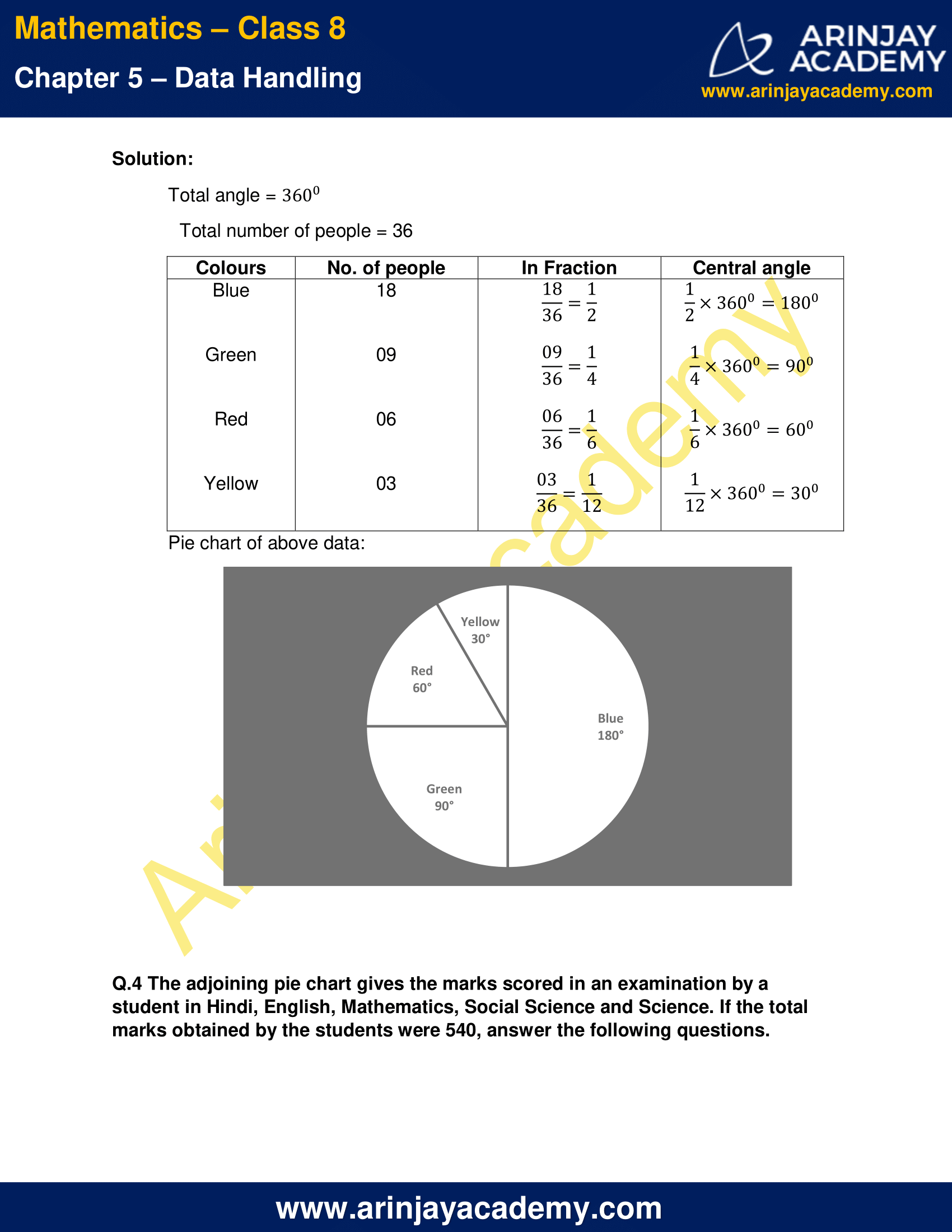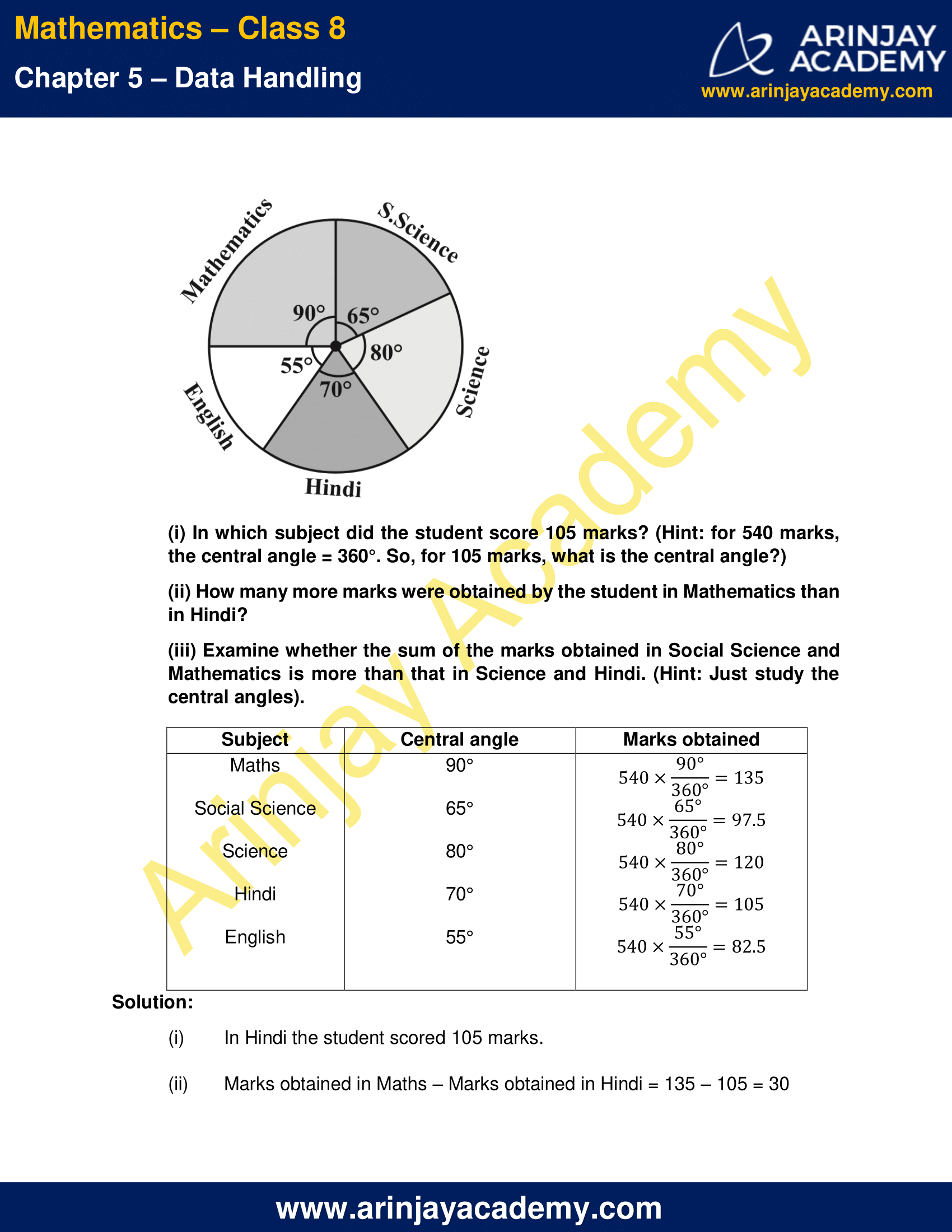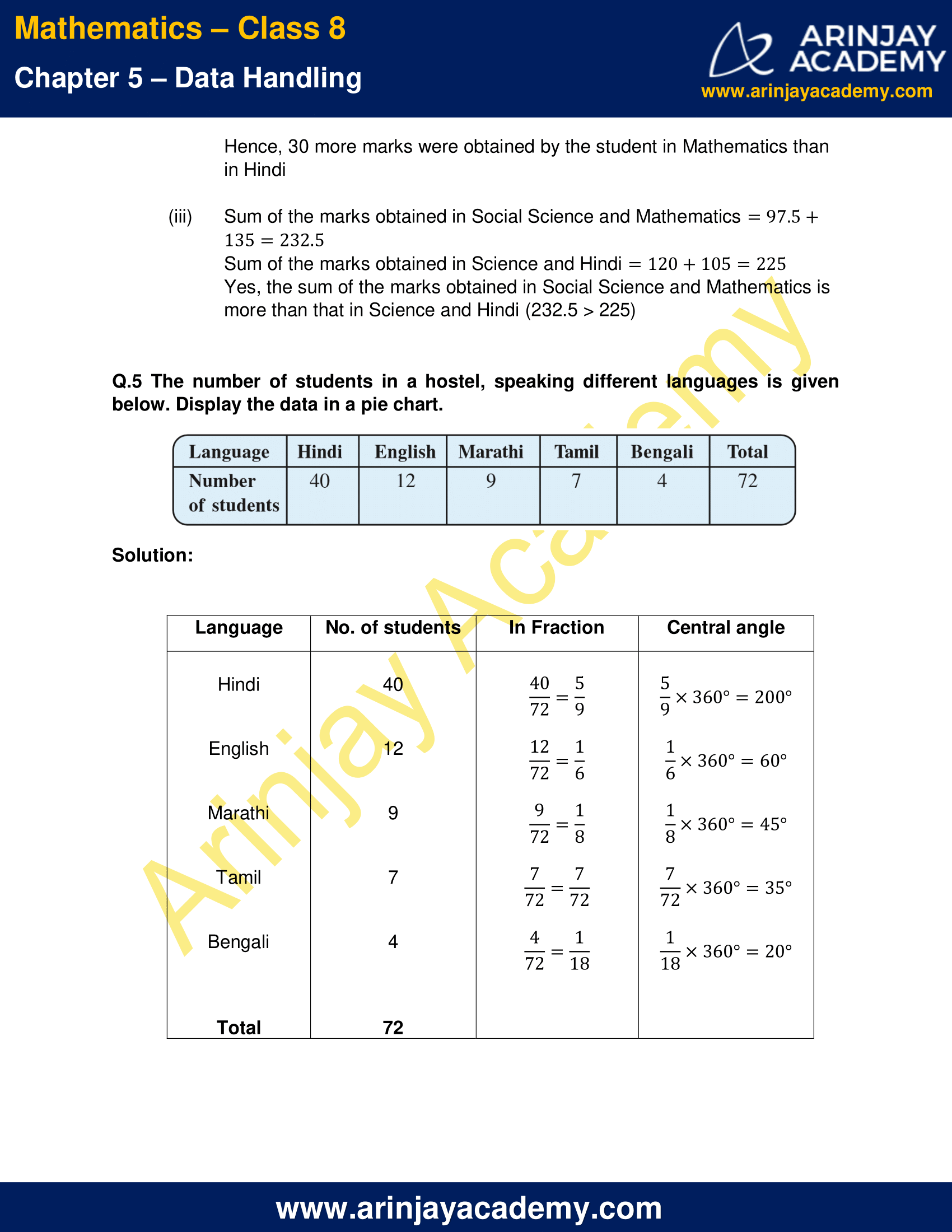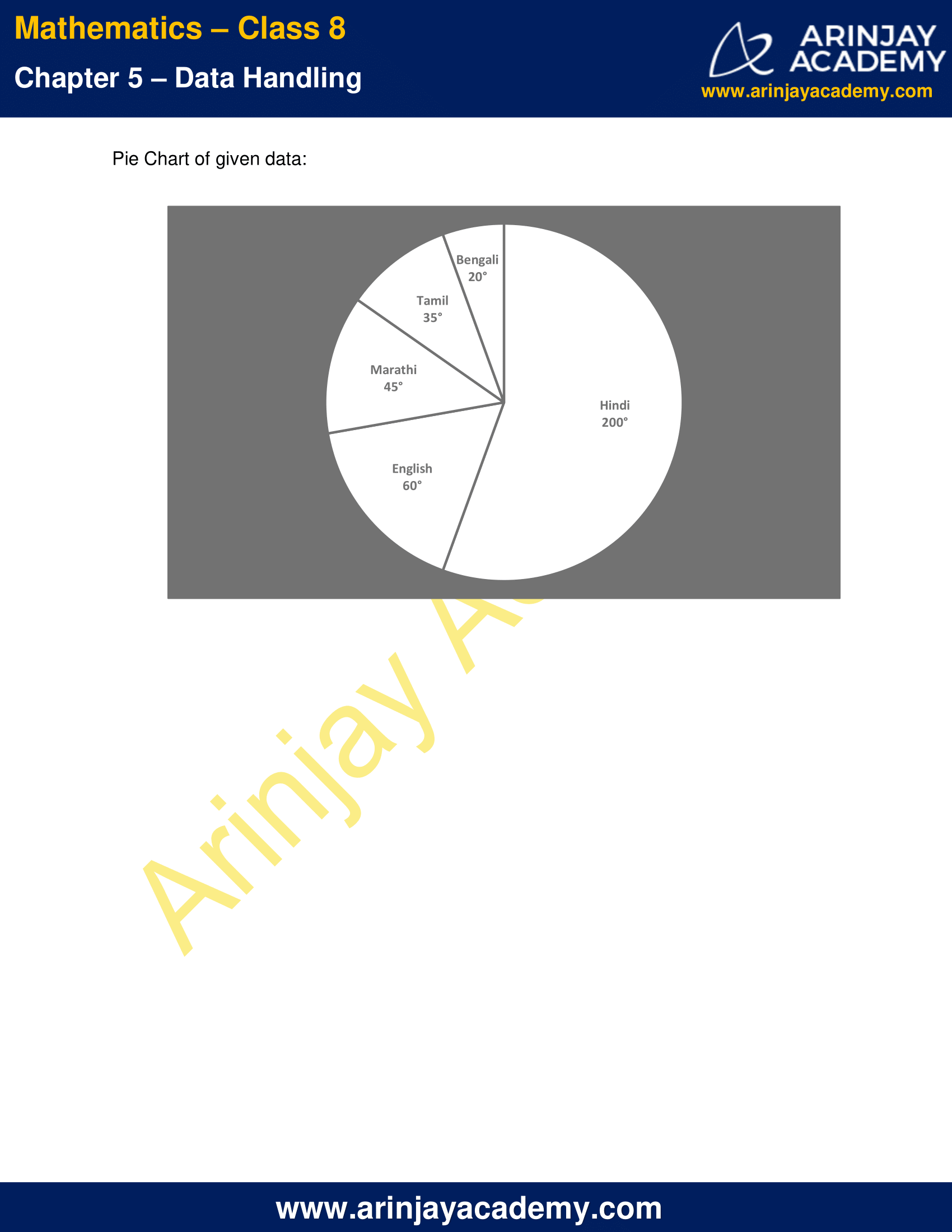NCERT Solutions for Class 8 Maths Chapter 5 Exercise 5.2

Q.1 A survey was made to find the type of music that a certain group of young people liked in a city. Adjoining pie chart shows the findings of this survey.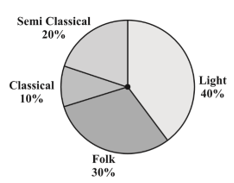From this pie chart answer the following:

(i)  If 20 people liked classical music, how many young people were surveyed?

(ii) Which type of music is liked by the maximum number of people?

(iii) If a cassette company were to make 1000 CD’s, how many of each type would they make?

Solution:

(i) Given, 20 people liked classical music
if 10% = 20
10 × 10 % = 20 × 10 = 200
Hence, 200 young people were surveyed

(ii) Light music is liked by maximum number of people.

(iii) CD’s of classical music = 1000 x (10/100) = 100 people
CD’s of Semi Classical = 1000 x (20/100) = 200 people
CD’s of Folk = 1000 x (30/100) = 300 people
CD’s of Light music = 1000 x (40/100) = 400 people

Q.2 A group of 360 people were asked to vote for their favourite season from the three seasons rainy, winter and summer.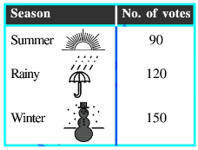(i) Which season got the most votes?
(ii) Find the central angle of each sector.
(iii) Draw a pie chart to show this information.

Solution:

(i) Winter season got the most votes

(ii) Central angle of summer season = (90°/360°) × 360° = 90°
Central angle of Rainy season = (120°/360°) × 360° = 120°
Central angle of Winter season = (150°/360°) × 360° = 150°

(iii) Pie chart: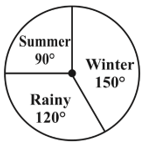Q.3 Draw a pie chart showing the following information. The table shows the colours preferred by a group of people.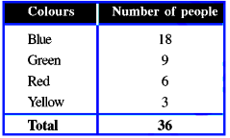Solution:

Total angle =  360°

Total number of people = 36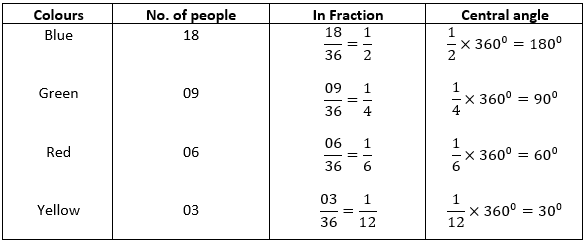Pie chart of above data: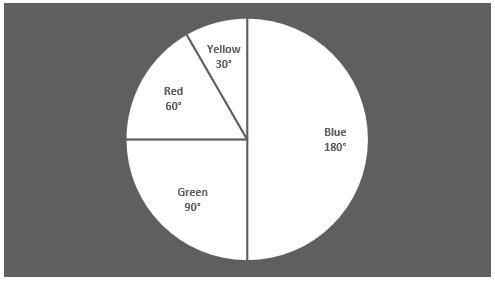Q.4 The adjoining pie chart gives the marks scored in an examination by a student in Hindi, English, Mathematics, Social Science and Science. If the total marks obtained by the students were 540, answer the following questions.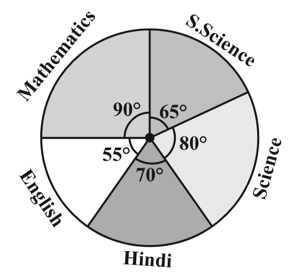(i) In which subject did the student score 105 marks? (Hint: for 540 marks, the central angle = 360°. So, for 105 marks, what is the central angle?)

(ii) How many more marks were obtained by the student in Mathematics than in Hindi?

(iii) Examine whether the sum of the marks obtained in Social Science and Mathematics is more than that in Science and Hindi. (Hint: Just study the central angles).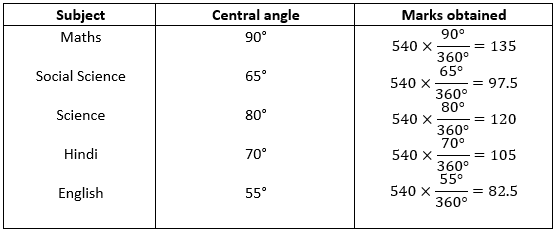Solution:

(i) In Hindi the student scored 105 marks.
(ii) Marks obtained in Maths – Marks obtained in Hindi = 135 – 105 = 30
Hence, 30 more marks were obtained by the student in Mathematics than in Hindi
(iii) Sum of the marks obtained in Social Science and Mathematics = 97.5+135 = 232.5
Sum of the marks obtained in Science and Hindi = 120+105 = 225
Yes, the sum of the marks obtained in Social Science and Mathematics is more than that in Science and Hindi (232.5 > 225)

Q.5 The number of students in a hostel, speaking different languages is given below. Display the data in a pie chart.Solution: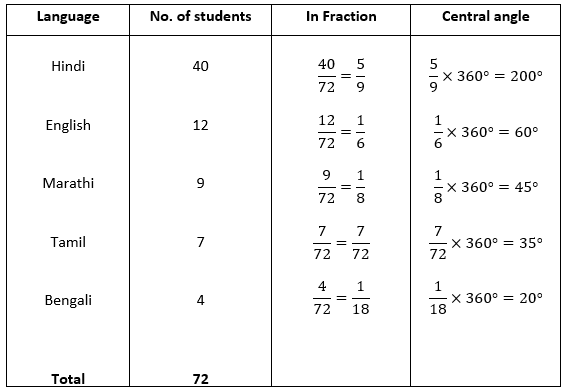Pie Chart of given data: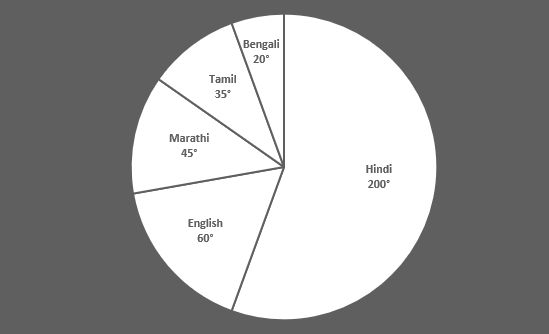The next Exercise for NCERT Solutions for Class 8 Maths Chapter 5 Exercise 5.3 – Data Handling can be accessed by